# DECT chip

Inputs are marked red, output green

Where no port names are given, the inputs are named A,B,... from left to right.

Flip Flop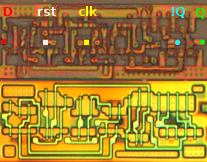(.xcf) 01: FlipFlop1(.xcf) 02: MUX-FlipFlop Q = ((A & B) | (~A & C))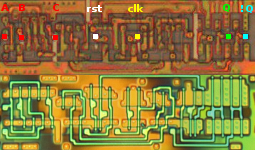(.xcf) 03: MUX-FlipFlop Q = ((A & B) | (~A & C))

Latch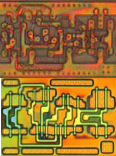(.xcf) 06: Latch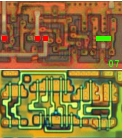(.xcf) 07: Latch

XOR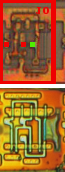(.psd) 10: XNOR Y = ~(A XOR B)(.psd) 11: XOR Y = (A XOR B)

OAI(.psd) 12: 22-OAI Y = ~((A | B) & (C | D))(.psd) 13: 21-OAI Y = ~(C & (A | B))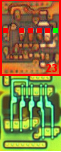(.psd) 23: 32-OAI Y = ~((A | B | C) & (D | E))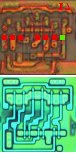(.psd) 17: 222-OAI Y = ~((D | C) & (B | E) & (A | F))(.xcf) 31: 31-OAI Y = ~((A | B | C) & D)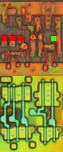(.xcf) 25: 21-OAI Y = ~((A | B) & C)

AOI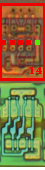(.psd) 14: 22-AOI Y = ~((B & C) | (A & D))(.psd) 18: 33-AOI Y = ~((A & B & C) | (D & E & F))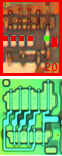(.psd) 20: 221-AOI Y = ~((B & C) | (A & D) | E)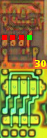(.xcf) 30: 21-AOI Y = ~(A | (B & C)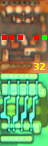(.xcf) 32: 31-AOI Y = ~((A & B & C) | D)

NOR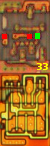(.xcf) 33: 2-NOR Y = ~(A | B)(.psd) 15: 2-NOR Y = ~(A | B)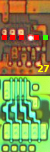(.xcf) 27: 4-NOR Y = ~(A | B | C | D)

NAND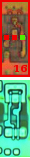(.psd) 16: 2-NAND Y = ~(A & B)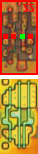(.psd) 22: 2-NAND Y = ~(~A & B)

MUX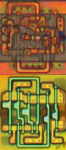(.xcf) 09: MUX Y = ~( (A AND B) OR (~A AND C))